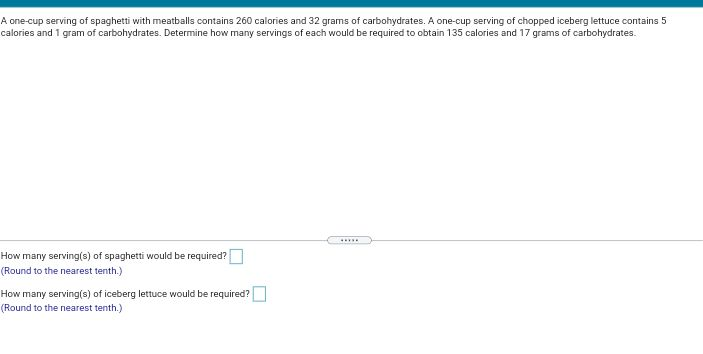### ¿Todavía tienes preguntas de matemáticas?

Pregunte a nuestros tutores expertos
Algebra
PreguntaA one-cup serving of spaghetti with meatballs contains $$260$$ calories and $$32$$ grams of carbohydrates. A one-cup serving of chopped iceberg lettuce contains $$5$$ calories and $$1$$ gram of carbohydrates. Determine how many servings of each would be required to obtain $$135$$ calories and $$17$$ grams of carbohydrates. How many serving(s) of spaghetti would be required? How many serving(s) of iceberg lettuce would be required?

$$0.5\\1$$